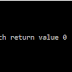Exponentiation is a mathematical operation, written as bn, involving two numbers, the base b and the exponent n. When n is a positive integer, exponentiation corresponds to repeated multiplication of the base: that is, bn is the product of multiplying n bases

• Using While Loop
• Through Function
• Through Recursive Function
• Using Pointer through Recursive Function

1. Using While Loop:
#include <stdio.h>
main() {
int pow, num,i = 1;
int result = 1;
printf ("Enter a number:");
scanf ("%d", &num);
printf ("Enter the power:");
scanf("%d", &pow);
while(i <= pow) {
result = result * num;
i++; }
printf("%d to the power %d is: %d", num, pow, result);  }
Output:
2. Through Function:
#include<stdio.h>
main() {
int power(int, int, int);
int pow, num;
int result = 1;
printf  ("Enter a number:");
scanf  ("%d", &num);
printf ("Enter the power:");
scanf("%d", &pow);
result = power(pow, num, result);
printf("%d to the power %d is: %d", num, pow, result); }
int power(int pow, int num, int result)  {
int i = 1;
while(i <= pow)  {
result = result * num;
i++;    }
return result;  }
Output:

3. Through Recursive Function:
#include <stdio.h>
main() {
int power(int, int);
int pow, num;
int result = 1;
printf ("Enter a number:");
scanf ("%d", &num);
printf ("Enter the power:");
scanf("%d", &pow);
result=power(num, pow);
printf("%d to the power %d is: %d", num, pow, result); }
int power(int num, int pow)  {
if(pow == 0)
return 1;
else
return num * power(num, pow-1);  }
Output:
4. Using Pointer through Recursive Function:
#include <stdio.h>
main() {
int power(int *, int *);
int pow, num;
int result = 1;
printf ("Enter a number:");
scanf ("%d", &num);
printf ("Enter the power:");
scanf("%d", &pow);
result=power(&num, &pow);
printf("%d to the power %d is: %d", num, pow, result); }
int power(int *num, int *pow) {
int a;
a = *pow -1;
if(*pow == 0)
return 1;
else
return (*num) * power(num, &a);  }
Output:

C Program to Calculate Power of a NumberReviewed by vijay pratap singh on May 18, 2017 Rating: 5